## Problem statement

A Pythagorean triplet is a set of three natural numbers,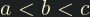, for which,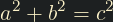For example,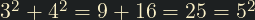. There exists exactly one Pythagorean triplet for which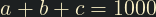. Find the product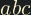## Thoughts

This could be easily brute-forced by iterating all pythagorean triples until we find the right one, but I think we can approach this more intelligently.

We have two known formulas that relate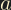,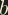, and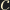: therefore we have a system of simultaneous equations. There are 3 unknowns so we don't have enough information to solve all 3 variables straight away, but this approach will get us part of the way there.is the 'unique' variable in this context, as it should be the sum of the other two. It doesn't matter which way aroundandare, they are the same in that respect.We have expressedin terms offor all values ofandsuch that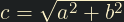and. We can plotagainst:Whilst it is true that all values ofresulting from any value ofwill satisfy our starting equations, we are told there is only one solutions where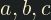are all integers greater than zero.

Unfortunately, finding integer solutions is non-trivial. This is a Diophantine equation, and a non-linear one at that. It is beyond my current mathematical ability to solve (I'm not sure if there is even a method to solve it).

I am, therefore, going to turn to a brute-force approach for solving this equation. We can easily see from the above plot that the upper bound foris 500 (above whichis negative), so that will be the upper bound for my search.

## Solution

Test every positive integer value ofin the above equation until we find one that yields an integer value foralso. We are told there is only one integer solution, so I will exit upon first finding one.

#include <math.h>
#include <stdio.h>
int main()
{
double a = 0;
double b = 1;

/* Iterate values of b looking for integer result a */
for (b = 1; b < 500; b++) {
a = (1000 * (b - 500)) / (b - 1000);

/* Break if a is an integer */
if (floor(a) == a) {
break;
}
}

/* Compute c from a^2 + b^2 = c^2 */
double c = sqrt((pow(a, 2) + pow(b, 2)));

printf("%f", a * b * c);
}